Contemporary Mathematics

# Chapter 12

12.1
1.
The vertices are p, q, r, s, and t. The edges are pq, pr, pt, qr, qs, qt, rs, and st.
12.2
1.
The pairs that are not adjacent are p and s, p and t, and r and t.
12.3
1.
Vertex q
12.4
1.
Graph Representing Common Boundaries Between Regions of Oahu
12.5
1.
The objects that are represented with vertices are poker players. An edge between a pair of vertices would indicate the two players competed against each other at table. This is best represented as a graph because players from the same table never meet a second time. Also, a player cannot compete against themself; so, there is no need for a loop.
12.6
1.
Graph E, 34
2.
Graph D, 18
3.
higher
12.7
1.
3
2.
Answers may vary. Two possible graphs are shown.
Graph 1: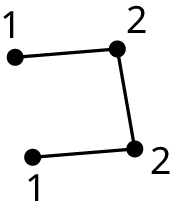Graph 2:12.8
1.
124,750 introductions
12.9
1.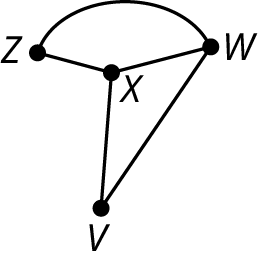12.10
1.
Triangle: (a, b, e or a, d, e)
Quadrilateral: (b, c, d, e or a, b, e, d)
Pentagon: (a, b, c, d, e)
12.11
1.
(B, D, C, E, F)
12.12
1.
186
12.13
1.
Graph C1 has 6 edges, but Graph C2 has 8.
Graph C1 has 4 vertices and Graph C2 has 5.
Graph C1 has no vertex of degree 4, but Graph C2 has one vertex of degree 4.
They do not have the same cycles. For example, Graph C2 has a pentagon cycle, but Graph C1 does not.
12.14
1.
• The total number of vertices in each graph is different. The Diamonds graph has 17 vertices while Dots graph has only 16.
• The degrees of vertices differ. The Diamonds graph has vertices of degrees 4 while the Dots graph does not.
• The graphs have different sizes of cyclic subgraphs. The Diamonds graph has 4 squares (4-cycles), while the Dots graph has 3 squares. Also, the Dots graph has 8-cycles while the Diamonds graph does not.
12.15
1.
Answers may vary. There are four possible isomorphisms:
• a − q, d − s, c − p, and b − r.
• a − p, d − s, c − q, and b − r.
• a − q, d − r, c − p, and b − s.
• a − p, d − r, c − q, and b − s.
12.16
1.
Caden is correct because Graph E could be untangled so that d is to the left of c and the edges bc and ad are no longer crossed. Maubi is incorrect because (a, b, c, d) is a quadrilateral in Graph E. Javier is incorrect because the portion of the graph he highlighted does not have 3 vertices and is not a triangle.
12.17
1.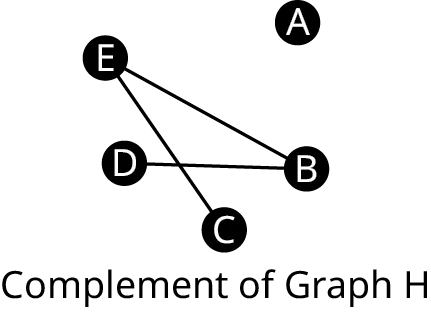12.18
1.
The degrees are 0. There are no adjacent vertices in Graph N since all vertices are adjacent in Graph M.
12.19
1.
c Only CVGBB is a walk.
12.20
1.
walk, path, and trail
2.
walk and a trail, but not a path
3.
none of these
12.21
1.
To fly from Palm Beach to any other city, you must take the flight from PBI to TPA. To return, you must take the flight from TPA to PBI. This means that the graph of any itinerary that begins and ends at PBI covers the same edge twice and is not a circuit.
2.
The degree of PBI is 1 meaning that there is only one edge connecting PBI to other parts of the graph.
12.22
1.
Yes. The chromatic number is 4 or less.
2.
3
3.
3 or 4
4.
Graphs 1 and 3
5.
Answers may vary. Here is an example of a 3-coloring: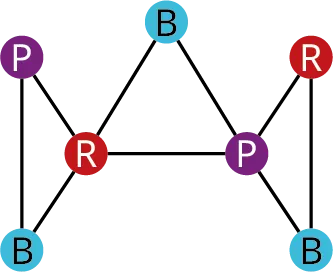12.23
1.
The chromatic number is 3. Here is a 3-coloring of the graph: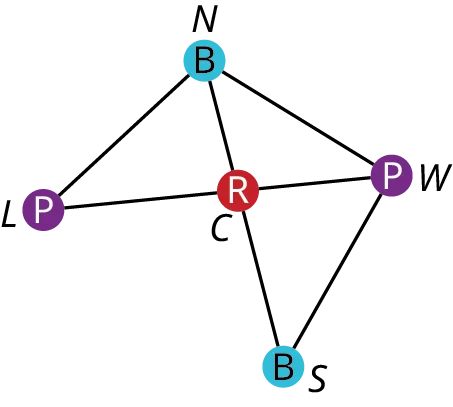12.24
1.
Graphs G, H, and I are connected; so, they each have a single component with the vertices {a, b, c, d}. Graph F is disconnected with two components, {a, c, d} and {b}. Graph J is disconnected with two components, {a, d} and {b, c}. Graph K is disconnected with three components, {a}, {b, d}, and {c}.
12.25
1.
Each pair of vertices would be connected by a path that was at most 6 edges long. So, the graph would be connected.
12.26
1.
The multigraph of the neighborhood is shown: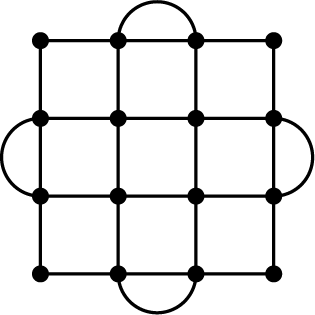2.
Yes, the graph is connected. It has only one component.
3.
There are four corner vertices of degree 2 and the remaining twelve vertices are all degree 4.
4.
Yes
5.
Yes, an Eulerian graph has an Euler circuit; so, it is possible.
12.27
1.
Graph X does not have an Euler circuit because it is disconnected.
2.
abgdfcgea
12.28
1.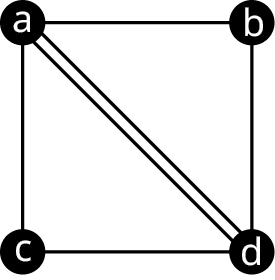12.29
1.
Yes.
2.
No. It doesn’t cover every edge.
3.
No. It is not a walk, because the vertices b and e are not adjacent.
12.30
1.
In a complete graph with three or more vertices, any three vertices form a triangle which is a cycle. Any edge in a graph that is part of a cycle cannot be a bridge so, there are no bridges in a complete graph. Also, any edge that is part of a triangle cannot be a local bridge because the removal of any edge of a triangle will leave a path of only two edges between the vertices of that edge. So, there are no bridges or local bridges in a complete graph.
12.31
1.
m
2.
qn, qt; qn bridge
3.
rs, st, tq, qn
4.
nm, np; nm,, bridge
5.
om, mp, pn, nm
6.
sqrstqnompnm
12.32
1.
The graph has Euler trails. They must begin and end at vertices u and v. An example is
vwxuzywu
12.33
1.
both
12.34
1.
$n! = 6! = 6 \cdot 5 \cdot 4 \cdot 3 \cdot 2 \cdot 1 = 720$ and $\left( {n - 1} \right)! = (6 - 1)! = 5! = 5 \cdot 4 \cdot 3 \cdot 2 \cdot 1 = 120$
12.35
1.
$5! = 5 \cdot 4 \cdot 3 \cdot 2 \cdot 1 = 120$
12.36
1.
120
12.37
1.
26
12.38
1.
No
2.
No
3.
Yes
12.39
1.
CABFDE
12.40
1.
pmonqtsr
2.
none
3.
none
12.41
1.
Hamilton path
2.
Euler trail
3.
neither
12.42
1.
brute force algorithm
12.43
1.

1. VWXYZV
2. VWXZYV
3. VWYXZV
4. VWYZXV
5. VWZXYV
6. VWZYXV
7. VXWYZV
8. VXWZYV
9. VXYWZV
10. VXYZWV (reverse of 6)
11. VXZWYV
12. VXZYWV (reverse of 4)
13. VYXWZV
14. VYXZWV (reverse of 5)
15. VYWXZV
16. VYWZXV (reverse of 11)
17. VYZXWV (reverse of 2)
18. VYZWXV (reverse of 8)
19. VZXYWV (reverse of 3)
20. VZXWYV (reverse of 15)
21. VZYXWV (reverse of 1)
22. VZYWXV (reverse of 7)
23. VZWXYV (reverse of 13)
24. VZWYXV (reverse of 9)
12.44
1.
The officer should travel from Travis Air Force Base to Beal Air Force Base, to Edwards Air Force Base, to Los Angeles Air Force Base, and return to Travis.
12.45
1.
DBEACFD; 550 min or 9 hrs 10 min.
12.46
1.
The star topology is a tree, and the tree topology is a tree. The ring topology and the mesh topology are not trees because they contain cycles.
12.47
1.
star topology
2.
tree topology
3.
none
12.48
1.
Vertices k, l, and m are in one component, and vertices g, h, i, and j are in the other.
2.
There are 6 vertices and 5 edges, which confirms Graph I is a tree, because the number of edges is one less than the number of vertices.
3.
12.49
1.
a True
2.
b False
3.
a True
4.
a True
12.50
1.
Answers may vary. Here are three possible spanning trees of Graph J:12.51
1.
The three edges must include one edge from list A and one pair of edges from list B.
List A: be, eh, hi, gi, bg
List B: ac and ad, ac and af, ac and cd, ac and cf, ad and af, ad and cd, ad and cf, af and cd, af and cf, or cd and cf.
12.52
1.
There are two possible minimum spanning trees, each with a total weight of 174: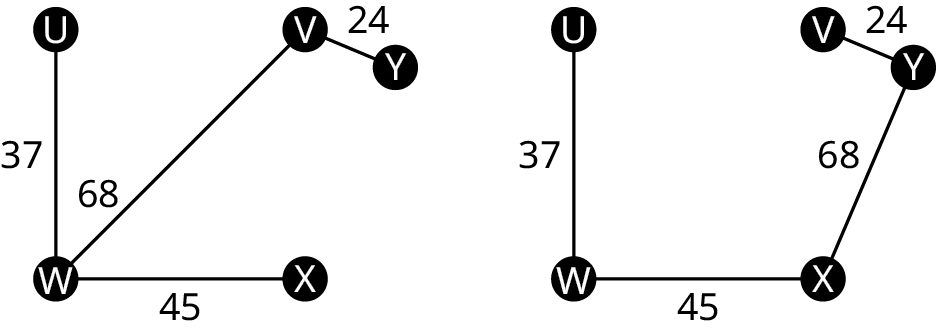1.
a True
2.
b False
3.
a True
4.
b False
5.
b False
6.
b False
7.
a True
8.
b False
9.
a True
10.
b
11.
a True
12.
The sum of the degrees is always double the number of edges, which means it has to be an even number, but 13 is odd.
13.
Yes. If n is the number of vertices in a complete graph, the number of edges is $\frac{{n(n - 1)}}{2} = \frac{n}{2}(n - 1)$ which is half the number of vertices times one less than the number of vertices.
14.
always true
15.
never true
16.
sometimes true
17.
never true
18.
always true
19.
sometimes true
20.
sometimes true
21.
never true
22.
sometimes true
23.
always true
24.
always true
25.
sometimes true
26.
always true
27.
sometimes true
28.
always true
29.
always true
30.
sometimes true
31.
always true
32.
sometimes true
33.
always true
34.
always true
35.
has no repeated egdes
36.
is closed
37.
has no repeated vertices
38.
has no repeated edges
39.
$n$
40.
at least
51.
edge
52.
Fleury’s
53.
circuit, trail
54.
components
55.
local bridge
56.
bridge
57.
vertex
58.
complete
59.
is
60.
is
61.
is not
62.
is
63.
is
64.
is not
65.
is not
66.
is not
67.
different from
68.
the same as
69.
different from
70.
different from
71.
b False
72.
b False
73.
b False
74.
a True
75.
a True
76.
b False
77.
b False
78.
b False
79.
a True
80.
b False
81.
b False
82.
a True
83.
a True
84.
b False
85.
b False
86.
a True
87.
a True
88.
a True
89.
a True
90.
b False
91.
b False
92.
a True
93.
a True
94.
a True
Order a print copy

As an Amazon Associate we earn from qualifying purchases.